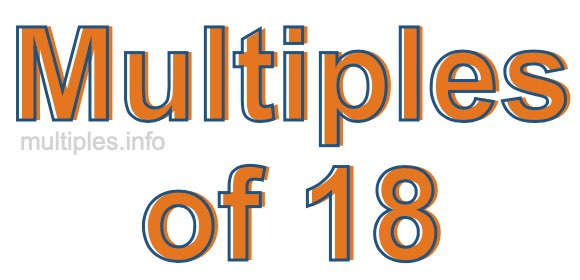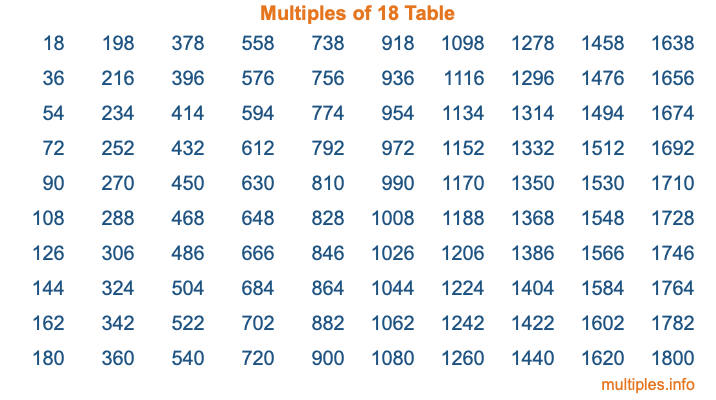Multiples of 18Welcome to the Multiples of 18 page. Here we will first teach you everything you will ever need to know about the multiples of 18, and then give you a study guide summary of everything we taught you to make sure you remember it all. Use this page to look up facts and learn information about the multiples of 18. This page will make you a multiples of eighteen expert!

Definition of Multiples of 18
Multiples of 18 are all the numbers that when divided by 18 equal an integer. Each of the multiples of 18 are called a multiple. A multiple of 18 is created by multiplying 18 by an integer.

Therefore, to create a list of multiples of 18, you start with 1 multiplied by 18, then 2 multiplied by 18, then 3 multiplied by 18, and so on for as long as you want. Thus, the list of the first five multiples of 18 is 18, 36, 54, 72, and 90. To see a larger list of multiples of 18, see the printable image of Multiples of 18 further down on this page. We also have a category where you can choose any nth multiple of 18.

Multiples of 18 Checker
The Multiples of 18 Checker below checks to see if any number of your choice is a multiple of 18. In other words, it checks to see if there is any number (integer) that when multiplied by 18 will equal your number. To do that, we divide your number by 18. If the the quotient is an integer, then your number is a multiple of 18.

Is  a multiple of 18?

Least Common Multiple of 18 and ...
A Least Common Multiple (LCM) is the lowest multiple that two or more numbers have in common. This is also called the smallest common multiple or lowest common multiple and is useful to know when you are adding our subtracting fractions. Enter one or more numbers below (18 is already entered) to find the LCM.

Check out our LCM Calculator if you need more details about the Least Common Multiple or if you need the LCM for different numbers for adding and subtraction fractions.

nth Multiple of 18
As we stated above, 18 is the first multiple of 18, 36 is the second multiple of 18, 54 is the third multiple of 18, and so on. Enter a number below to find the nth multiple of 18.

th multiple of 18

Multiples of 18 vs Factors of 18
18 is a multiple of 18 and a factor of 18, but that is where the similarities end. All postive multiples of 18 are 18 or greater than 18. All positive factors of 18 are 18 or less than 18.

Below is the beginning list of multiples of 18 and the factors of 18 so you can compare:

Multiples of 18: 18, 36, 54, 72, 90, etc.

Factors of 18: 1, 2, 3, 6, 9, 18

As you can see, the multiples of 18 are all the numbers that you can divide by 18 to get a whole number. The factors of 18, on the other hand, are all the whole numbers that you can multiply by another whole number to get 18.

It's also interesting to note that if a number (x) is a factor of 18, then 18 will also be a multiple of that number (x).

Multiples of 18 vs Divisors of 18
The divisors of 18 are all the integers that 18 can be divided by evenly. Below is a list of the divisors of 18.

Divisors of 18: 1, 2, 3, 6, 9, 18

The interesting thing to note here is that if you take any multiple of 18 and divide it by a divisor of 18, you will see that the quotient is an integer.

Multiples of 18 Table
Below is an image of the first 100 multiples of 18 in a table. The table is in chronological order, column by column. The first column has the first ten multiples of 18, the second column has the next ten multiples of 18, and so on.The Multiples of 18 Table is also referred to as the 18 Times Table or Times Table of 18. You are welcome to print out our table for your studies.

Negative Multiples of 18
Although not often discussed or needed in math, it is worth mentioning that you can make a list of negative multiples of 18 by multiplying 18 by -1, then by -2, then by -3, and so on, to get the following list of negative multiples of 18:

-18, -36, -54, -72, -90, etc.

Multiples of 18 Summary
Below is a summary of important Multiples of 18 facts that we have discussed on this page. To retain the knowledge on this page, we recommend that you read through the summary and explain to yourself or a study partner why they hold true.

There are an infinite number of multiples of 18.

A multiple of 18 divided by 18 will equal a whole number.

18 divided by a factor of 18 equals a divisor of 18.

The nth multiple of 18 is n times 18.

The largest factor of 18 is equal to the first positive multiple of 18.

18 is a multiple of every factor of 18.

18 is a multiple of 18.

A multiple of 18 divided by a divisor of 18 equals an integer.

18 divided by a divisor of 18 equals a factor of 18.

Any integer times 18 will equal a multiple of 18.

Multiples of a Number
Here you can get the multiples of another number, all with the same attention to detail as we did for multiples of 18 on this page.

Multiples of
Multiples of 19
Did you find our page about multiples of eighteen educational? Do you want more knowledge? Check out the multiples of the next number on our list!